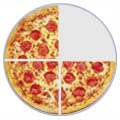# Proper FractionsA Proper Fraction has a top number less than its bottom number 38 (Three-Eighths)

## Examples

 38 14 1415 45

See how the top number is smaller than the bottom number in each example? That makes it a Proper Fraction.

## Three Types of Fractions

There are three types of fraction:## Fractions

A Fraction (such as 3/8) has two numbers:

NumeratorDenominator

The top number is the Numerator, it is the number of parts you have.
The bottom number is the Denominator, it is the number of parts the whole is divided into.### Example: 3/4 means:

• We have 3 parts
• Each part is a quarter (1/4) of a whole

So we can define the three types of fractions like this:

Proper Fractions: The numerator is less than the denominator Examples: 1/3, 3/4, 2/7 The numerator is greater than (or equal to) the denominator Examples: 4/3, 11/4, 7/7 A whole number and proper fraction together Examples: 1 1/3, 2 1/4, 16 2/5

## Proper Fractions

So, a proper fraction is just a fraction where the numerator (the top number) is less than the denominator (the bottom number). Here are some examples of proper fractions:1/2 1/4 3/8 (One-Half) (One-Quarter) (Three-Eighths)